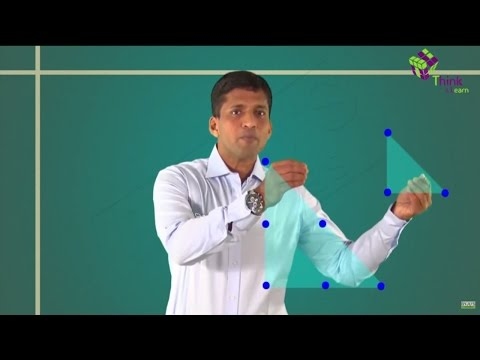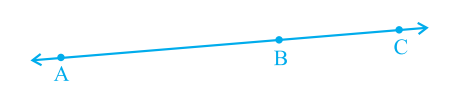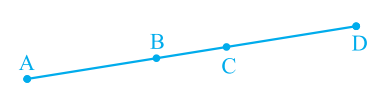# Introduction to Euclid’s Geometry Class 9 Notes: Chapter 5

## Introduction to Euclid’s Geometry

Geometry is a branch of mathematics that includes the study of different shapes and sizes we observe in our day-to-day life. The Greek mathematician Euclid introduced geometry with a new definition, axioms and postulates. In the introduction to Euclid’s geometry chapter, we will learn Euclid’s approach to geometry and how it is related to present geometry.

## What Is Euclid’s Geometry?

The word ‘geometry’ comes from the Greek words ‘geo’, which means the ‘earth’, and ‘metron’, which means ‘to measure’. Euclidean geometry is a mathematical system attributed to Euclid, a teacher of mathematics in Alexandria, Egypt. Euclid gave us an exceptional idea regarding the basic concepts of geometry, in his book named “Elements”.

Euclid listed 23 definitions in his book. Some important points are mentioned below:

• A line is an endless length.
• A point has no dimension (length, breadth and width).
• A line which lies evenly with the points on itself is a straight line.
• Points are the ends of a line.
• A surface is that which has breadth and length only.
• A plane surface is a surface which lies evenly with straight lines on itself.
• Lines are the edges of a surface.Euclid realized that a precise development of geometry must start with the foundations. Euclid’s axioms and postulates are still studied for a better understanding of geometry.

Know more: Euclidean Geometry

## Euclid’s Postulates

The five postulates put forward by Euclid are:

• Postulate 1: A straight line may be drawn from any one point to any other point.
• Postulate 2: A terminated line can be produced indefinitely.
• Postulate 3: A circle can be drawn with any centre and any radius.
• Postulate 4: All right angles are equal to one another.
• Postulate 5: If a straight line falling on two straight lines makes the interior angles on the same side of it taken together less than two right angles, then the two straight lines, if produced indefinitely, meet on that side on which the sum of angles is less than two right angles.

## Euclid’s Axioms

The seven axioms by Euclid are given below.

• Things which are equal to the same thing are equal to one another.
• If equals are added to equals, the wholes are equal.
• If equals are subtracted from equals, the remainders are equal.
• Things which coincide with one another are equal to one another.
• The whole is greater than the part.
• Things which are double of the same things are equal to one another.
• Things which are halves of the same things are equal to one another.

## Theorem

According to this theorem, two distinct lines cannot have more than one point in common.
Proof: Say, there are two lines ‘l’ and ‘m’ that have one common point.
Now, let us suppose, ‘l’ and ‘m’ have two common points, say A and B. So, two lines, l and m, pass through two distinct points A and B. But the assumption contradicts with the axiom that only one line can pass through two distinct points. Hence, the assumption we made is wrong and the two lines ‘l’ and ‘m’ have only one common point.

## Equivalent Versions of Euclid’s Fifth Postulate

(i) ‘For every line l and for every point P not lying on l, there exists a unique line ‘m’ passing through P and parallel to l’.

(ii) Two distinct intersecting lines cannot be parallel to the same line.

Know more: Euclid’s Fifth Postulates

## Video Lesson

#### For more information on Introduction To Euclid’s Geometry, watch the below video### Important Questions

Q.1) If A, B and C are 3 points on a line, and B lies between A and C, prove that AB + BC = AC.Q.2) Prove that an equilateral triangle can be formed on any given line segment.

Q.3) If AC = BD, then prove that AB = CD.Students of class 9 can refer the introduction to Euclid’s geometry Class 9 notes to prepare for their exam more effectively. Stay tuned with BYJU’S and get detailed notes of all concepts of Class 9 mathematics.

## Frequently Asked Questions on CBSE Class 9 Maths Notes Chapter 5 – Introduction to Euclids Geometry

Q1

### What is Euclids geometry?

Euclidean geometry is the study of plane and solid figures on the basis of axioms and theorems employed by the Greek mathematician Euclid.

Q2

### What is an infinity point?

An ideal mathematical point in projective geometry that preserves the magnitudes of all angles of a transformed plane is called an infinity point.

Q3

### Who was Alexandrian?

He was a Greek mathematician and engineer who was active in his native city of Alexandria, Roman Egypt.